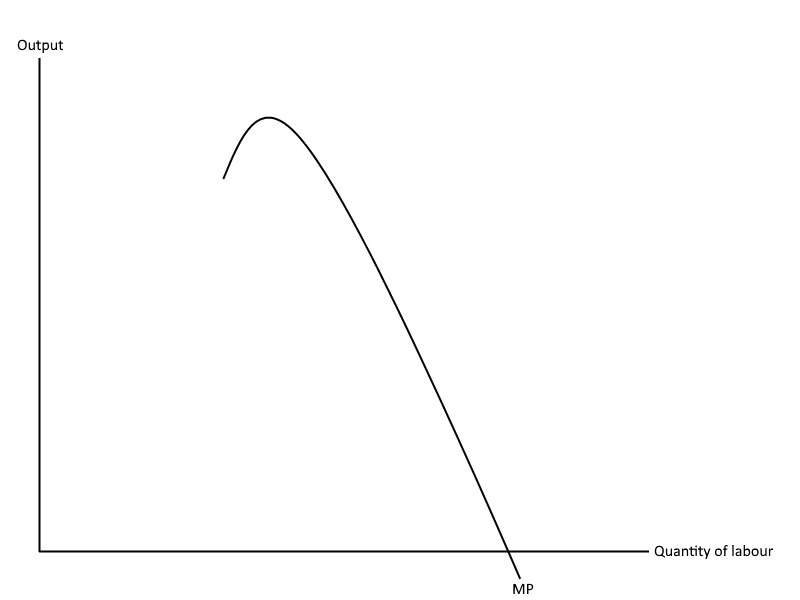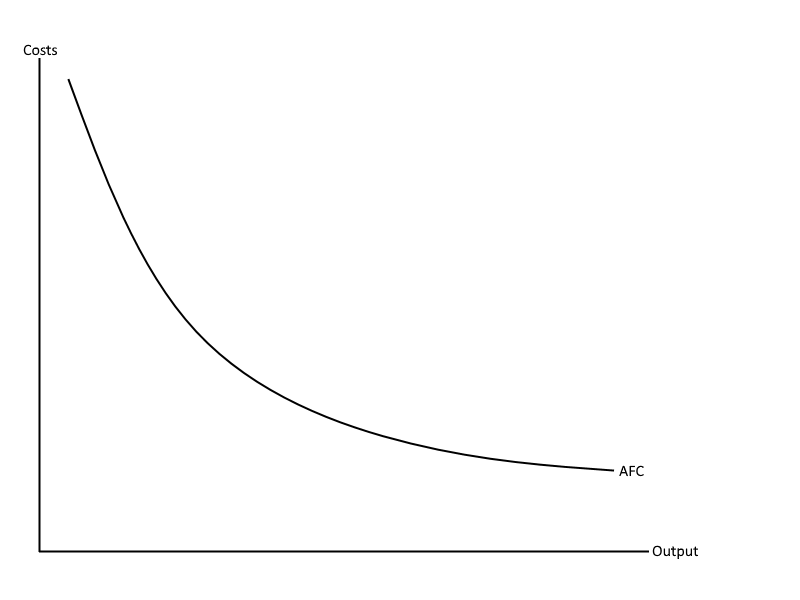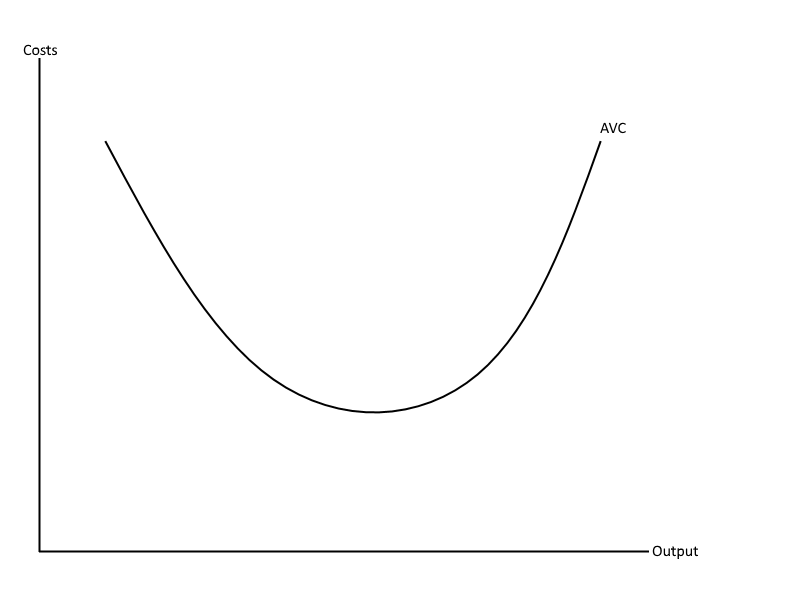# Costs

## Formulae

Total cost = total fixed cost x total variable cost

Total variable costs = variable cost x quantity

Average (total) cost = total cost ÷ quantity

Average fixed cost = total fixed cost ÷ quantity

Average variable cost = total variable cost ÷ quantity

Marginal cost = change in cost ÷ change in quantity

## Law of diminishing marginal productivity

In the short run at least one factor of production is fixed, whereas in the long run all factors of production are variable. For example, in the short run firms it is difficult for firms to respond to increases in demand through expansion. This is due to the large amount of money and time it takes to buy land. Therefore, we assume that this factor is usually fixed in the short term. However in the long run, firms are likely to have the time to build new buildings and expand, meaning that land is now variable.

The law of diminishing marginal utility occurs when an increase in the quantity of variable factors e.g. labour are added to a fixed factor e.g. land. This can be shown by the following example.

 Labour Total product Marginal product 0 0 0 1 3 3 2 10 7 3 14 4 4 15 1 5 12 -3When the first unit of labour is added, marginal productivity increases from 0 to 3. When the second unit of labour is added to the fixed factor of production (land), the marginal product increases even more. This is because workers are now able to specialize on certain tasks, thus increasing productivity further. Furthermore, by the time that the second unit of labour is added, the first unit of labour will have improved at doing their job through repetition, thus also increasing their productivity. However, when the third worker is added, marginal productivity decreases due to the fact that workers are constrained to the small piece of land that they have to work on, meaning the fourth worker is likely to make working conditions cramped which decreases productivity per worker. By the time the firth worker is added, the fixed factor of production (land) is becoming over utilized. This means that adding workers actually causes total productivity to decrease rather than increase.

An example of this could be found in a restaurant. Having a few workers there allows specialization e.g. one worker specializes on deserts and another on main courses etc. At the beginning there is extra space that is currently being underutilized and therefore extra workers are able to be added. However, after a certain point, adding too many workers will cause total productivity to fall as workers are bumping into each other or may have to wait for other workers to finish using the microwave or oven before they can use it. Therefore, in order to get past the constraint of limited land, they will have to expand in the long run.

## Average fixed costsAlthough total fixed costs stay the same, average fixed costs decrease as a firm’s quantity of goods/services produced increases. This makes sense when looking at the averaged fixed costs formula which is: Total fixed costs/Quantity. For example, if a firm’s total fixed costs were £1000 and the firm increase their quantity of goods/services produced from 100 to 200 the firm’s average fixed costs will decrease from £10 to £5. This explains the shape of the curve, which decreases as output increases.

## Average variable costsUnlike average fixed costs, average variable costs change with output e.g. raw material costs. For example, as demand increases for a firm’s product, they will have to increase the amount of raw materials that they use to make the products in order to meet the increase in demand.

The shape of the average variable cost curve can be explained through the law of diminishing marginal returns. As the quantity of labour increases so will total productivity due to labour specialization and as this increases average variable costs will decrease. However after a certain point, fixed factors of production such as land become over utilized and labour becomes constraint to the limited space. This causes output per worker to decrease and therefore average variable costs will start to increase again, thus explaining the shape of the average variable cost curve.

## Total costs

Fixed costs = Costs that don’t vary with output e.g. RentTotal fixed costs stay the same regardless of the output of the firm, therefore TFC is represented by a straight horizontal line. Just like the average variable cost curve, the total variable cost curve is also influenced by… Read more